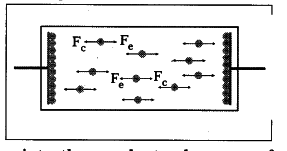# How does a battery work ? Explain

1. When a conducting wire is connected to the terminals of the battery, a potential difference is created between the ends of the conductor.
2. This potential difference sets up an electrical field throughout the conductor. The conductor contains large number of electrons.
3. The electrons near the positive terminal of battery are attracted by it and start to move towards positive terminal.
4. As a result, the amount of positive charge on the plate decreases.
5. So, the electric force F _{ e } becomes weaker
than chemical force F _{c} and the chemical force pulls negative ions from the positive plate (anode) and makes them move towards the negative plate (cathode).
6. The negative terminal pushes one electron into the conductor because of stronger repulsion between negative terminal and negative ion.
7. Hence, the total number of electrons in the conductor remains constant during the current flow.
8. The above process continues till equilibrium is attained between electric force [F _{ e }] and chemical force [F _{c}].